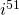# 9.9 Complex Numbers (Optional)

Throughout history, there has been the need for a number that has a zero value (0), numbers smaller than zero (negatives), numbers between integers (fractions), and numbers between fractions (irrational numbers). This need arises when trying to take an even root, such as the square root of a negative number. To accomplish this, mathematicians have created what are termed imaginary and complex numbers.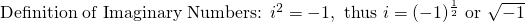From this, notice that: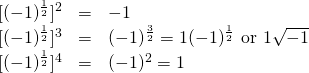The nature of imaginary numbers generates the following repeating pattern: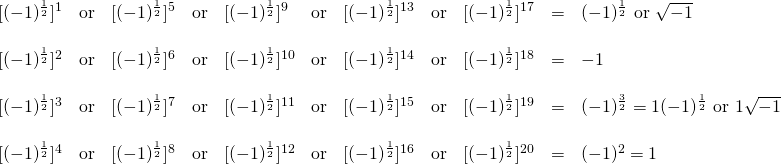Using the symbolto represent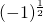, the above pattern can be rewritten as: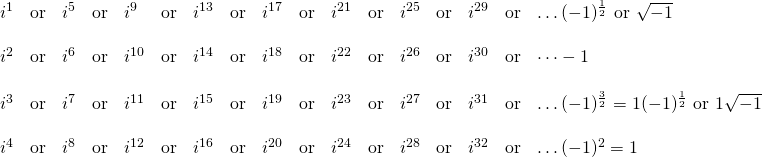Notice: This is a repeating pattern of the exponent 4.

Examples of imaginary numbers include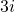,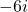,and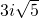.

Complex numbers are ones that contains both real and imaginary parts, such as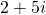.

With this algebraic creation, even powered roots of negative numbers are no longer undefined and should now be able to do basic operations with any root having negatives.

First, consider exponents on imaginary numbers, where the easiest way to reduce them is to divide the exponent by 4.

Example 9.91

Reduce the imaginary number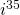.

First, take the power 35 and divide it by 4.

35 ÷ 4 yields 8¾

The 8 is irrelevant in this solution, sinceis the same as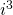.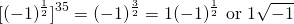Example 9.9.2

Reduce the imaginary number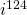.

First, take the power 124 and divide it by 4.

124 ÷ 4 yields 31

The 8 becomes irrelevant in this solution, sinceis the same as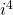or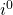.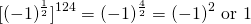When performing operations such as adding, subtracting, multiplying, and dividing with complex radicals, work withjust like it was handled previous polynomials. This means, when adding and subtracting complex numbers, simply add or combine like terms.

Example 9.9.3

Add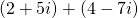.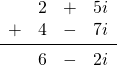Example 9.9.4

Subtract and add the following complex numbers: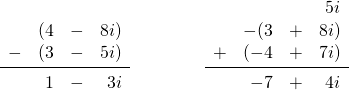Multiplying with complex numbers is the same as multiplying with polynomials, with one exception:  simplify the final answer so that there are no exponents on.

Example 9.9.5

Multiply the following complex numbers: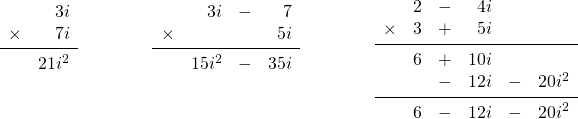The last step in these solutions is to simplify allexponents.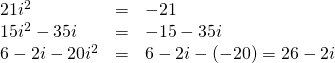Dividing complex numbers also has one thing to be careful of. If you haveorin the denominator, then there is a radical in the denominator, which means that it will need to be rationalized. This is done using the same process used to rationalize denominators with square roots.

Example 9.9.6

Divide the following complex numbers: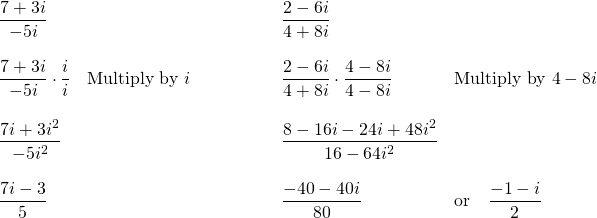# Questions

Simplify.

1.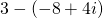2.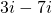3.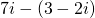4.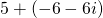5.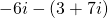6.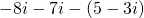7.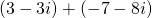8.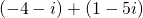9.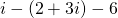10.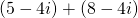11.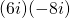12.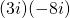13.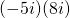14.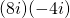15.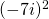16.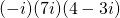17.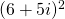18.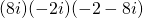19.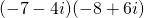20.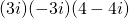21.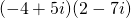22.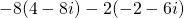23.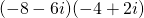24.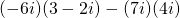25.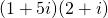26.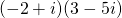27.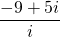28.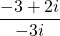29.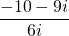30.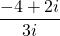31.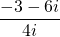32.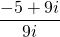33.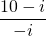34.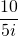35.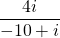36.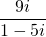37.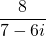38.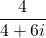39.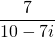40.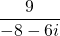41.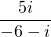42.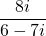43.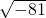44.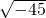45.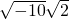46.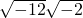47.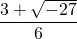48.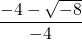49.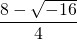50.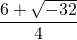51.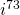52.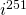53.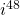54.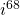55.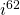56.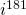57.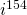58.